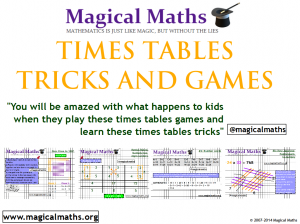# Hints Tricks and Tips on Learning Times Tables! :: UPDATED ::time image picture clipart

Learning times tables

Parents of toddlers and primary school teachers  are always looking for better ways in learning times tables. The first thing is to get a times tables chart and make sure that this is visible as times table learning aid and a way in which the learner can practice. Over the years I have taught the times tables to hundreds of pupils and to be honest some methods are great and others are not. The success of the pedagogy of teaching times tables all depends on the learner, time of day and the mood that they are in.

After learning their times tables get your pupils to learn how Japanese pupils learn to multiply numbers or if you are looking for an effective Maths game have a look at this learning times table maths game. How about challenging the more able students and telling them the secret of learning their 13 to 20 times tables.

I have summarised a few ways that teachers, parents and pupils can use to help in the learning of time tables. Comments are FREE! If you have any methods that you would like to add to the list, please add your comments below.

Hints Tricks Tips on Learning your 2 times table

• You should add the number to itself, for example 2 × 8 = 8 + 8

Hints Tricks Tips on Learning your 5 times table

• The last digit will always be 5,0,5,0,..,
• The answer is always half of 10 time table , for example: 5 x 6 = half of 10 x 6 = half of 60 = 30
• The answer is half the number times 10, for example 5 x 6 = 10 x 3 = 30

Hints Tricks Tips on Learning your 6 times table

• If you multiply 6 by an even number, they both end in the same digit. For example: 6×2=12, 6×4=24, 6×6=36

Hints Tricks Tips on Learning your 9 times table

• The answer is 10× the number minus the number. For Example: 9×6 = 10×6 – 6 = 60-6 = 54
• The last digit of the answer is always goes 9,8,7,6, and so on
• The sum of the answer’s digits is always 9. For example: 9 × 5 = 45 and 4 + 5 = 9. (This does not work with 9 × 11 = 99)

Hints Tricks Tips on Learning your 10 times table

• Put a zero after the number you multiplying 10 by

Hints Tricks Tips on Learning your 11 times table

• For the answers up to 9 x 11  repeat the digit. For example 4 x 11 = 44
• For the answers 10 x 11 to 18 x 11: write the sum of the digits between the digits. For example 15 x 11 = 1(1+5)5 = 165) – this works for any two digit number, but if the sum of the digits is more than 9, carry the one.

Hints Tricks Tips on Learning your 12 times table

• The answer is 10 × the number add 2 × number

If you enjoyed using the resource above then make sure to check out the games and tricks available to help develop, practice and learn times tables skills.Tagged with: ,
###### 3 comments on “Hints Tricks and Tips on Learning Times Tables! :: UPDATED ::”
1.nancy wimbush says:

I have worked with junior and MS learners in ‘open ended’ problem solving learning and discovery/inquiry framework. Kids love to share existing knowledge and discovering and sharing these ‘tricks’. Thanks for your post. Great stuff:)

2.Raz Well says:

So exhilarating! Please keep me posted with your new methods to empower my learners. By the way,I am a Mathematics and Physical Sciences teacher.

Thank you!
Raz Well

•magicalmaths says:

Will do. Thanks!